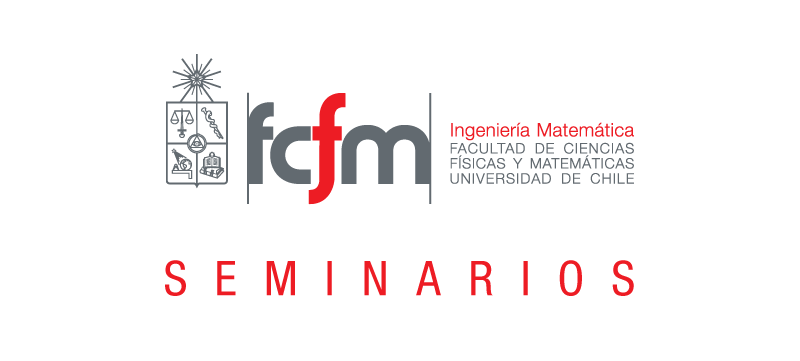Seleccionar páginaLunes 07 de Agosto 2023 a las 12:00 hrs.,Sala de Seminarios, 5th floor, Beauchef 851

Speaker: Ricardo Freire, Postdoc ANID en el DIM

Title: Energy decay for classes of nonlocal dispersive equations.

Abstract:

We consider the long-time dynamics of large solutions to a special class of evolution equations. Using virial techniques, we describe regions of space where every solution in a suitable Sobolev space must decay to zero along sequences of times.

Moreover, in the case of interior regions, we prove decay for a sequence of times. The classes of nonlocal dispersive equations which we will treat are as follows:

{∂t u + Lαu + u∂x u = 0, x, t ∈ R,u(x, 0) = u0(x),

where α > 0, and the operator Lα is the Fourier multiplier operator by a real-valued odd function belonging to (C 1(R) ∩ C ∞(R∗)).

These classes contain, in particular, the following equations: the fractional KdV, Benjamin-Ono and the Intermediate Long Wave, for example.

Venue: Online via Zoom

El link para acceder a la reunión de zoom es el siguiente:

https://reuna.zoom.us/j/87947528106?pwd=SkJaMGd6aVlDTzMyb1kvUk9talhJQT09